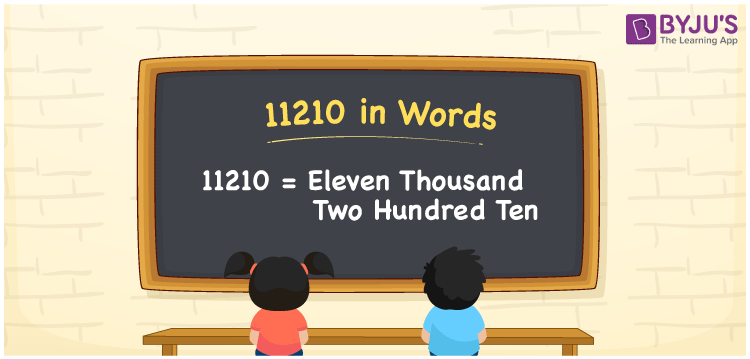# 11210 in Words

The number 11210 in words is written as “Eleven Thousand Two Hundred Ten”. The number 11210 is used to count things or it represents some value. The conversion of numbers to words can be done with the help of a place value system. In this article, let us discuss how to write 11210 in words with its procedure in detail.

 11210 in Words: Eleven Thousand Two Hundred Ten. Eleven Thousand Two Hundred Ten in Numerical Form: 11210.

## 11210 in English Words## How to Write 11210 in Words?

The following is the place value table for the number 11210. Here, 11 is in the thousands place, 2 is in the hundreds place, 1 is in the tens place and 0 is in one’s place.

 Ten – Thousands Thousands Hundreds Tens Ones 1 1 2 1 0

The expanded form of 11210 is as follows:

= 1× Ten thousand + 1 × Thousand + 2 × Hundred + 1 × Ten + 0 × One

= 1 × 10000 + 1 × 1000 + 2 × 100 + 1 × 10

= 10000 + 1000 + 200 + 10

= 11210

= Eleven thousand two hundred ten

Hence, 11210 in words is eleven thousand two hundred ten.

11210 in words – Eleven thousand two hundred ten

Is 11210 an odd number? – No

Is 11210 an even number? – Yes

Is 11210 a perfect square number? – No

Is 11210 a perfect cube number? – No

Is 11210 a prime number? – No

Is 11210 a composite number? – Yes

## Frequently Asked Questions on 11210 in Words

Q1

### How to write 11210 in English words?

11210 in words can be written as eleven thousand two hundred ten.

Q2

### Simplify 11000 + 210, and express in words.

Simplifying 11000 + 210, we get 11210. Hence, 11210 in words is eleven thousand two hundred ten.

Q3

### Is 11210 an odd number?

No, 11210 is not an odd number.

Test your Knowledge on 11210 in Words